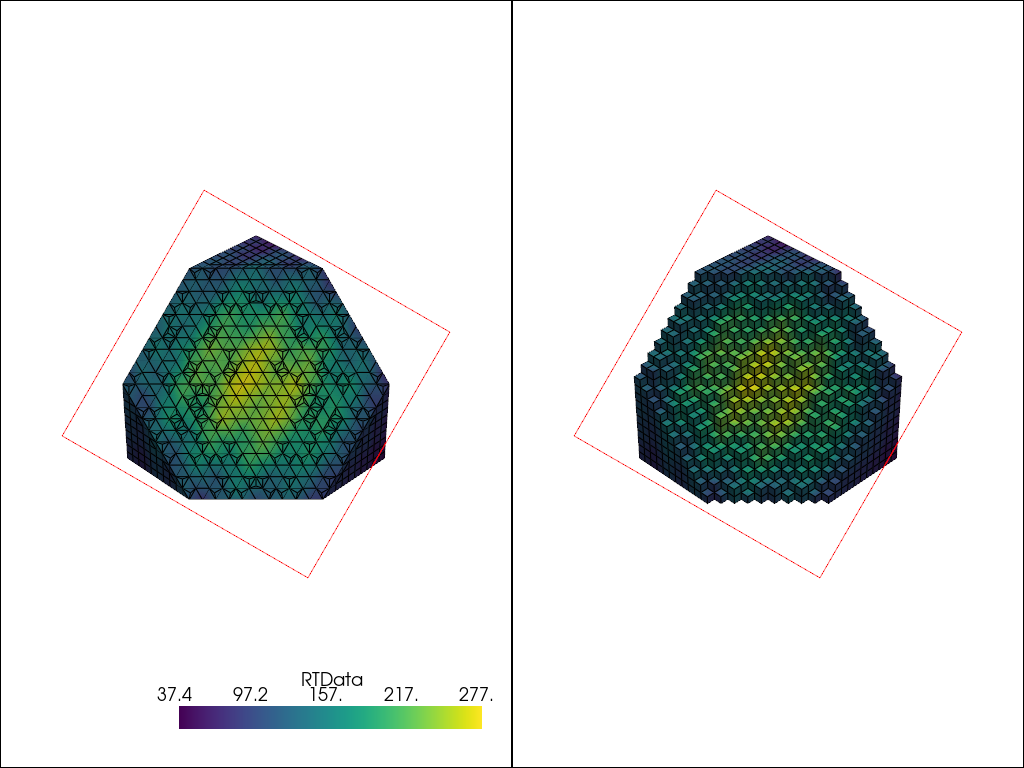# Clipping with Planes & Boxes#

Clip/cut any dataset using using planes or boxes.

```import pyvista as pv
from pyvista import examples
```

## Clip with Plane#

Clip any dataset by a user defined plane using the `pyvista.DataSetFilters.clip()` filter

```dataset = examples.download_bunny_coarse()
dataset
```
PolyDataInformation
N Cells1000
N Points872
N Strips0
X Bounds-1.316e-01, 1.802e-01
Y Bounds-1.205e-01, 1.877e-01
Z Bounds-1.430e-01, 9.851e-02
N Arrays1
NameFieldTypeN CompMinMax
NormalsPointsfloat323-9.998e-019.977e-01

```help(dataset.clip)
```
```Help on method clip in module pyvista.core.filters.data_set:

clip(normal='x', origin=None, invert=True, value=0.0, inplace=False, return_clipped=False, progress_bar=False, crinkle=False) method of pyvista.core.pointset.PolyData instance
Clip a dataset by a plane by specifying the origin and normal.

If no parameters are given the clip will occur in the center
of that dataset.

Parameters
----------
normal : tuple(float) or str, default: 'x'
Length 3 tuple for the normal vector direction. Can also
be specified as a string conventional direction such as
``'x'`` for ``(1,0,0)`` or ``'-x'`` for ``(-1,0,0)``, etc.

origin : tuple(float), optional
The center ``(x,y,z)`` coordinate of the plane on which the clip
occurs. The default is the center of the dataset.

invert : bool, optional
Flag on whether to flip/invert the clip.

value : float, optional
Set the clipping value along the normal direction.
The default value is 0.0.

inplace : bool, optional

return_clipped : bool, optional
Return both unclipped and clipped parts of the dataset.

progress_bar : bool, optional
Display a progress bar to indicate progress.

crinkle : bool, optional
Crinkle the clip by extracting the entire cells along the
clip. This adds the ``"cell_ids"`` array to the ``cell_data``
attribute that tracks the original cell IDs of the original
dataset.

Returns
-------
pyvista.PolyData or tuple(pyvista.PolyData)
Clipped mesh when ``return_clipped=False``,
otherwise a tuple containing the unclipped and clipped datasets.

Examples
--------
Clip a cube along the +X direction.  ``triangulate`` is used as
the cube is initially composed of quadrilateral faces and
subdivide only works on triangles.

>>> import pyvista as pv
>>> cube = pv.Cube().triangulate().subdivide(3)
>>> clipped_cube = cube.clip()
>>> clipped_cube.plot()

Clip a cube in the +Z direction.  This leaves half a cube
below the XY plane.

>>> import pyvista as pv
>>> cube = pv.Cube().triangulate().subdivide(3)
>>> clipped_cube = cube.clip('z')
>>> clipped_cube.plot()

See :ref:`clip_with_surface_example` for more examples using this filter.
```

Perform a clip with a Y axis normal

```clipped = dataset.clip('y', invert=False)
clipped
```
PolyDataInformation
N Cells427
N Points248
N Strips0
X Bounds-1.316e-01, 1.326e-01
Y Bounds3.360e-02, 1.877e-01
Z Bounds-1.430e-01, 8.721e-02
N Arrays1
NameFieldTypeN CompMinMax
NormalsPointsfloat323-9.927e-019.977e-01

```p = pv.Plotter()
p.camera_position = [(0.24, 0.32, 0.7), (0.02, 0.03, -0.02), (-0.12, 0.93, -0.34)]
p.show()
```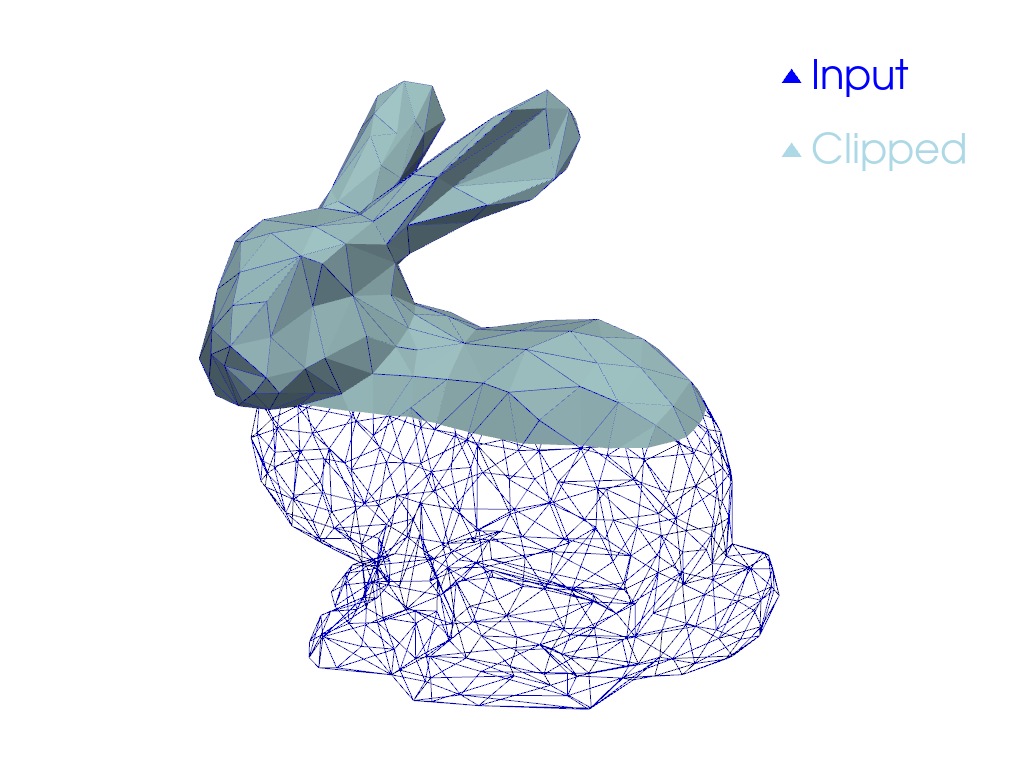## Clip with Bounds#

Clip any dataset by a set of XYZ bounds using the `pyvista.DataSetFilters.clip_box()` filter.

```dataset = examples.download_office()
```
```help(dataset.clip_box)
```
```Help on method clip_box in module pyvista.core.filters.data_set:

clip_box(bounds=None, invert=True, factor=0.35, progress_bar=False, merge_points=True, crinkle=False) method of pyvista.core.pointset.StructuredGrid instance
Clip a dataset by a bounding box defined by the bounds.

If no bounds are given, a corner of the dataset bounds will be removed.

Parameters
----------
bounds : tuple(float), optional
Length 6 sequence of floats: (xmin, xmax, ymin, ymax, zmin, zmax).
Length 3 sequence of floats: distances from the min coordinate of
of the input mesh. Single float value: uniform distance from the
min coordinate. Length 12 sequence of length 3 sequence of floats:
a plane collection (normal, center, ...).
:class:`pyvista.PolyData`: if a poly mesh is passed that represents
a box with 6 faces that all form a standard box, then planes will
be extracted from the box to define the clipping region.

invert : bool, optional
Flag on whether to flip/invert the clip.

factor : float, optional
If bounds are not given this is the factor along each axis to
extract the default box.

progress_bar : bool, optional
Display a progress bar to indicate progress.

merge_points : bool, optional
If ``True`` (default), coinciding points of independently
defined mesh elements will be merged.

crinkle : bool, optional
Crinkle the clip by extracting the entire cells along the
clip. This adds the ``"cell_ids"`` array to the ``cell_data``
attribute that tracks the original cell IDs of the original
dataset.

Returns
-------
pyvista.UnstructuredGrid
Clipped dataset.

Examples
--------
Clip a corner of a cube.  The bounds of a cube are normally
``[-0.5, 0.5, -0.5, 0.5, -0.5, 0.5]``, and this removes 1/8 of
the cube's surface.

>>> import pyvista as pv
>>> cube = pv.Cube().triangulate().subdivide(3)
>>> clipped_cube = cube.clip_box([0, 1, 0, 1, 0, 1])
>>> clipped_cube.plot()

See :ref:`clip_with_plane_box_example` for more examples using this filter.
```

Clip the dataset with a bounding box defined by the values in `bounds` (xmin, xmax, ymin, ymax, zmin, zmax)

```bounds = [2, 4.5, 2, 4.5, 1, 3]
clipped = dataset.clip_box(bounds)
clipped
```
UnstructuredGridInformation
N Cells38690
N Points9438
X Bounds1.000e-02, 4.500e+00
Y Bounds1.000e-02, 4.500e+00
Z Bounds1.000e-02, 2.500e+00
N Arrays2
NameFieldTypeN CompMinMax
scalarsPointsfloat321-3.870e+007.186e-01
vectorsPointsfloat323-6.729e-014.289e-01

```p = pv.Plotter()
p.show()
```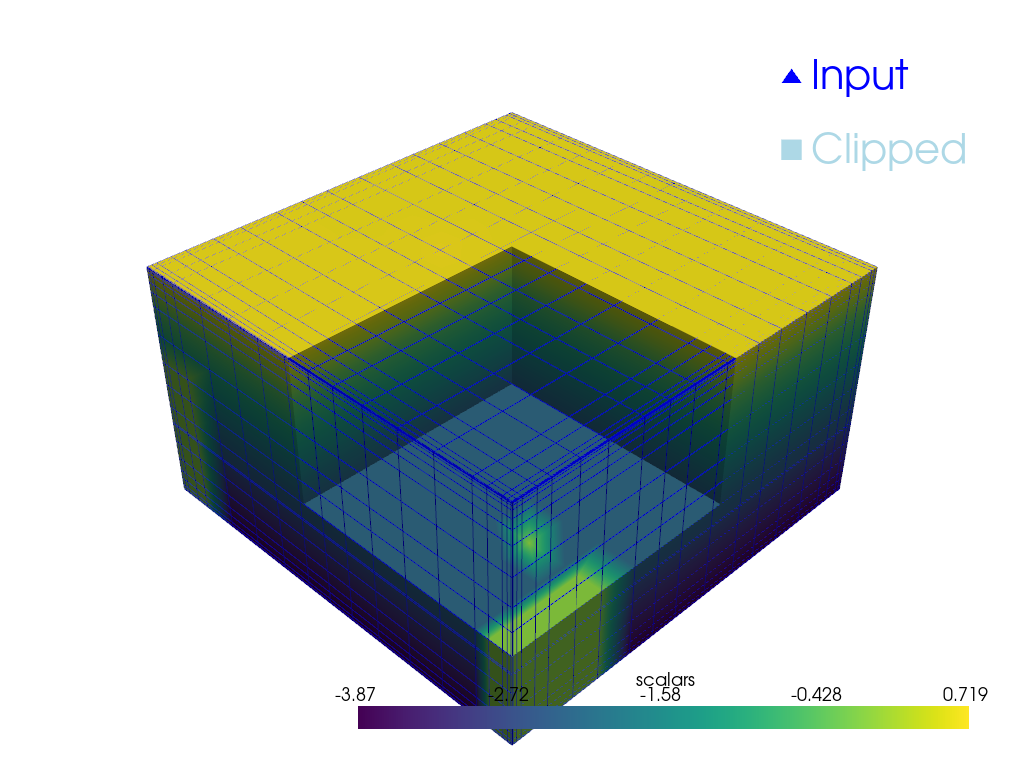## Clip with Rotated Box#

Clip any dataset by an arbitrarily rotated solid box using the `pyvista.DataSetFilters.clip_box()` filter.

```mesh = examples.load_airplane()

# Use `pv.Box()` or `pv.Cube()` to create a region of interest
roi = pv.Cube(center=(0.9e3, 0.2e3, mesh.center), x_length=500, y_length=500, z_length=500)
roi.rotate_z(33, inplace=True)

p = pv.Plotter()
p.show()
```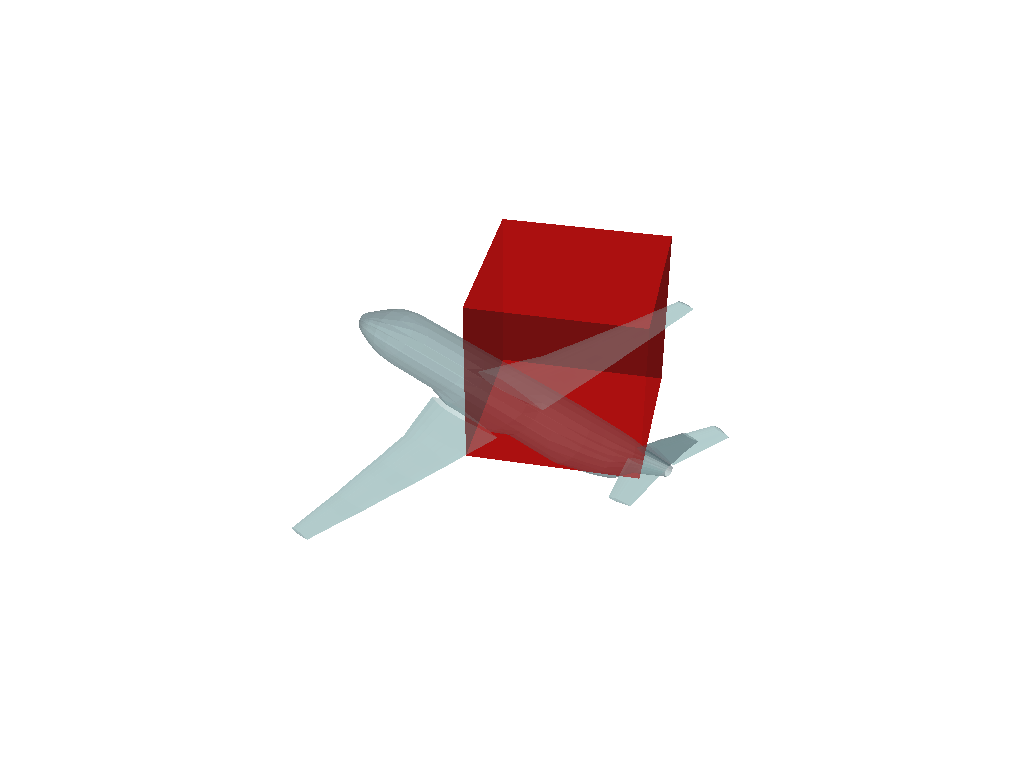Run the box clipping algorithm with the defined box geometry

```extracted = mesh.clip_box(roi, invert=False)

p = pv.Plotter(shape=(1, 2))
p.subplot(0, 1)
p.view_isometric()
p.show()
```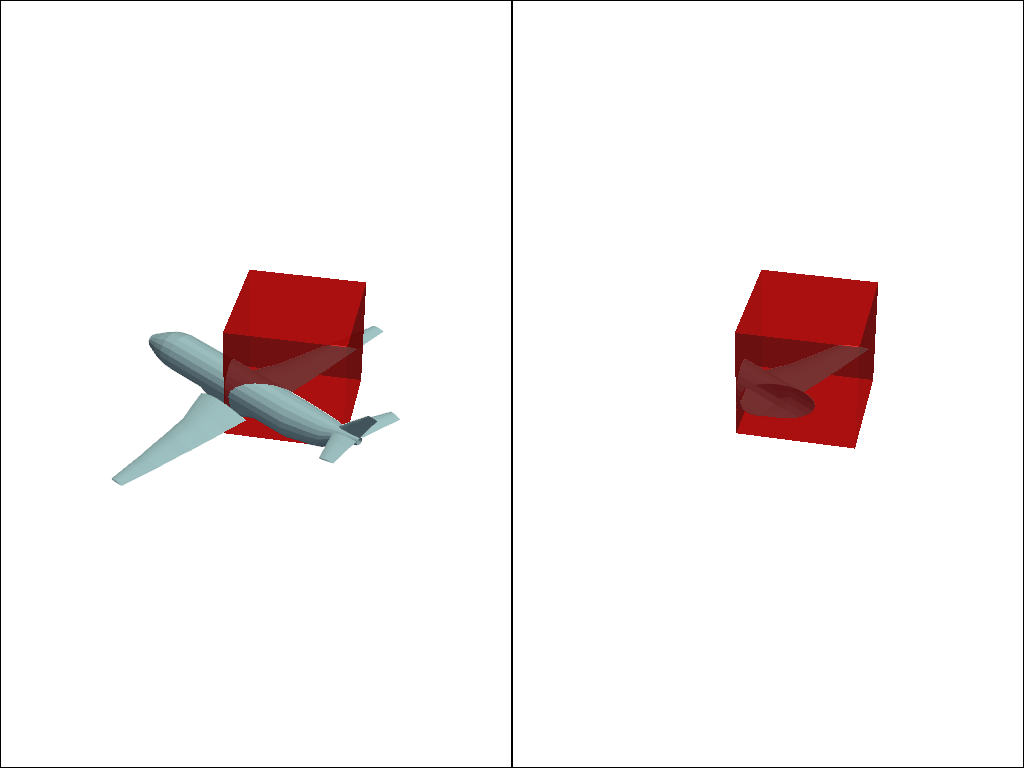## Crinkled Clipping#

Crinkled clipping is useful if you don’t want the clip filter to truly clip cells on the boundary, but want to preserve the input cell structure and to pass the entire cell on through the boundary.

This option is available for `pyvista.DataSetFilters.clip()`, `pyvista.DataSetFilters.clip_box()`, and `pyvista.DataSetFilters.clip_sruface()`, but not available when clipping by scalar in `pyvista.DataSetFilters.clip_scalar()`.

```# Input mesh
mesh = pv.Wavelet()
```

Define clipping plane

```normal = (1, 1, 1)
plane = pv.Plane(i_size=30, j_size=30, direction=normal)
```

Perform a standard clip

```clipped = mesh.clip(normal=normal)
```

Perform a crinkled clip to compare

```crinkled = mesh.clip(normal=normal, crinkle=True)
```

Plot comparison

```p = pv.Plotter(shape=(1, 2))